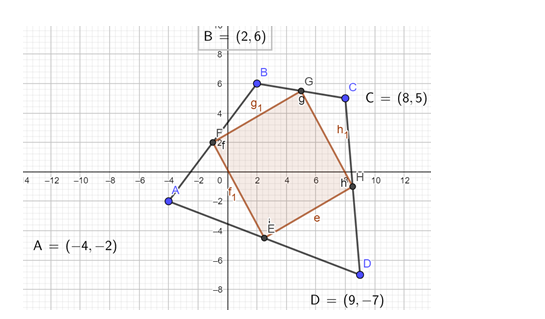# The vertices of a quadrilateral are A`
Question:

The vertices of a quadrilateral are A(-4, -2), B(2, 6), C(8, 5) and D(9, -7). Using slopes, show that the midpoints of the sides of the quad. ABCD from a parallelogram.

Solution:The vertices of the given quadrilateral are A(-4,-2) B(2, 6), C(8, 5) and D(9, -7)

The mid point of a line $A\left(x_{1}, y_{1}\right)$ and $B\left(x_{2}, y_{2}\right)$ is found out by $\left(\frac{x_{1}+x_{2}}{2}, \frac{y_{1}+y_{2}}{2}\right)$

Now midpoint of $A B=\left(\frac{-4+2}{2}, \frac{-2+6}{2}\right)=(-1,2)$

The midpoint of $B C=\left(\frac{2+8}{2}, \frac{6+5}{2}\right)=(5,5.5)$

The midpoint of $C D=\left(\frac{8+9}{2}, \frac{5-7}{2}\right)=(8.5,-1)$

Midpoint of $D A=\left(\frac{-4+9}{2}, \frac{-2-7}{2}\right)=(2.5,-4.5)$

So now we have four points

$P(-1,2), Q(5,5.5), R(8.5,-1), S(2.5,-4.5)$

slope $=\left(\frac{y_{2}-y_{1}}{x_{2}-x_{1}}\right)$

Slope of $\mathrm{PQ}=\left(\frac{5.5-2}{5+1}\right)=\frac{3.5}{6}=\frac{7}{12}$

Slope of $Q R=\left(\frac{-1-5.5}{8.5-5}\right)=\frac{-6.5}{3.5}=\frac{-1.3}{0.7}=\frac{-13}{7}$

Slope of RS $=\left(\frac{-4.5+1}{2.5-8.5}\right)=\frac{-3.5}{-6}=\frac{7}{12}$

Slope of SP $=\left(\frac{-4.5-2}{2.5+1}\right)=\frac{-6.5}{3.5}=\frac{-13}{7}$

Now we can observe that slope of $P Q=R S$ and slope of $Q R=S P$

Which shows that line $P Q$ is parallel to $R S$ and line $Q R$ is parallel to $S P$

Also, the product of two adjacent lines is not equal to $-1$

Therefore PQRS is a parallelogram.